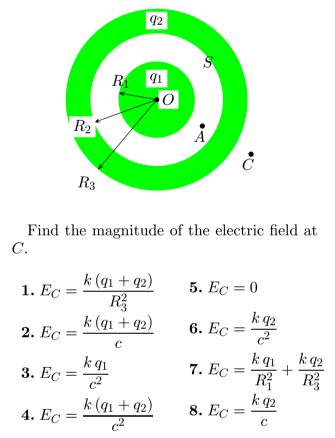# Problem: A solid conducting sphere of radius R1 and total charge q1 is enclosed by a conducting shell with an inner radius R2 and outer radius R3 and total charge q2. OA = a and OC = c.

###### Problem Details

A solid conducting sphere of radius R1 and total charge q1 is enclosed by a conducting shell with an inner radius R2 and outer radius R3 and total charge q2. OA = a and OC = c.